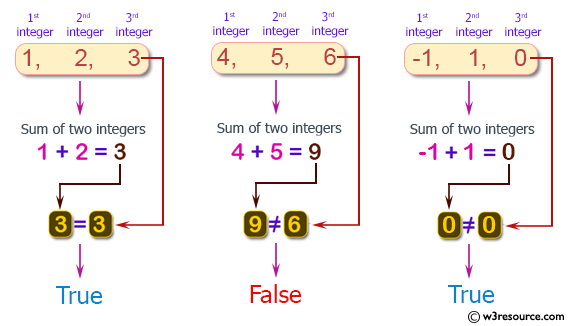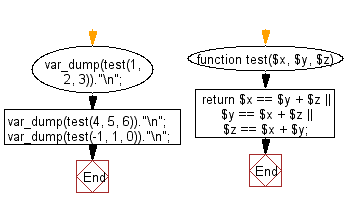﻿ PHP Exercises: Check if it is possible to add two integers to get the third integer from three given integers - w3resource# PHP Exercises: Check if it is possible to add two integers to get the third integer from three given integers

## PHP Basic Algorithm: Exercise-46 with Solution

Write a PHP program to check if it is possible to add two integers to get the third integer from three given integers.

Sample Solution:

PHP Code :

``````<?php
function test(\$x, \$y, \$z)
{
return \$x == \$y + \$z || \$y == \$x + \$z || \$z == \$x + \$y;
}

var_dump(test(1, 2, 3))."\n";
var_dump(test(4, 5, 6))."\n";
var_dump(test(-1, 1, 0))."\n";
``````

Sample Output:

```bool(true)
bool(false)
bool(true)
```

Pictorial Presentation:Flowchart:PHP Code Editor:

What is the difficulty level of this exercise?

﻿

## PHP: Tips of the Day

\$_REQUEST: This SuperGlobal Variable is used to collect data submitted by a HTML Form

Example:

This code save in a php file and run in the browser.

```<!DOCTYPE html>
<html>
<body>

<form method="post" action="<?php echo \$_SERVER['PHP_SELF'];?>">
NAME: <input type="text" name="user">
<button type="submit">SUBMIT</button>
</form>
<?php
if (\$_SERVER["REQUEST_METHOD"] == "POST") {
\$name = htmlspecialchars(\$_REQUEST['user']);
if(empty(\$name)){
echo "Name is empty";
} else {
echo \$name;
}
}
?>
</body>
</html>
```

Output:

```Owen
```